## Section29.4Superposition of Electric Fields

Here, we want to address the question: How do you get net electric field if multiple fields are acting at the same time?

Recall that net force on an object is a vector sum of the forces on that object. Now, if several charges act on a test charge $Q\text{,}$ with forces $\vec F_1\text{,}$ $\vec F_2\text{,}$ $\cdots\text{,}$ $\vec F_N\text{,}$ then, net electric force on $Q$ will be

\begin{equation*} \vec F_e = \vec F_1 + \vec F_2 + \cdots + \vec F_N. \end{equation*}

Now, if we divide both sides by the test charge, we will get

\begin{equation*} \frac{\vec F_e}{Q} = \frac{\vec F_1}{Q} + \frac{\vec F_2}{Q} + \cdots + \frac{\vec F_N}{Q}. \end{equation*}

Each of the $\vec F_i/Q$ is actually, the electric field from the corresponding source, which we can denote by $\vec E_i\text{.}$ One the left is the net electric force divided by the test charge, so, we get the net electric field there, which we denote by $\vec E_P\text{.}$ Therefore, we find that, just like forces, electric fields also add vectorially to give the net electric field.

\begin{equation} \vec E_P = \vec E_1 + \vec E_2 + \cdots + \vec E_N. \label{eq-e-field-super-1}\tag{29.4.1} \end{equation}

This result is called superposition of electric fields. We will work out examples of this principle below.

Four charged plates are arranged with positive plates facing the negative plates. The charges on the opposing plates along horizontal direction produce an electric field of magnitude $E_1 = 3 \text{ N/C}$ in the horizontal direction as shown in the figure, and the other two plates produce $E_2 = 4 \text{ N/C}$ in the vertical direction. What is the electric field of all charges?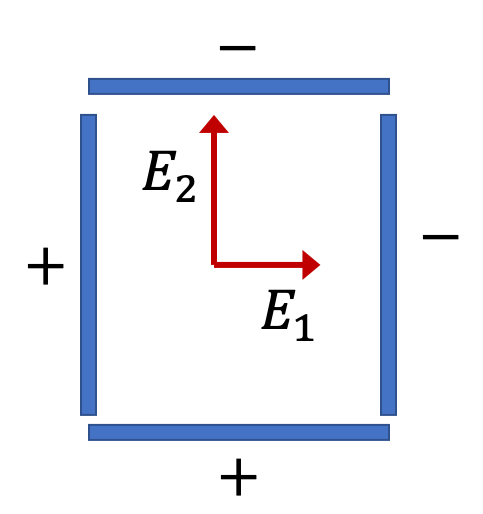Hint

$5\text{ N/C}$ at $53^{\circ}$ counterclockwise from the horizontal field direction.

Solution

We need to add the electric fields vectorially. Since the fields are already in the perpendicular directions, it is easy to work out the magnitude by Pythagora's theorem.

\begin{equation*} E = \sqrt{ 3^2 + 4^2} = 5\text{ N/C}. \end{equation*}

The direction can be given by the counterclockwise angle with respect to the horizontal direction,

\begin{equation*} \theta = \tan^{-1}\left( \dfrac{4}{3} \right) = 53^{\circ}. \end{equation*}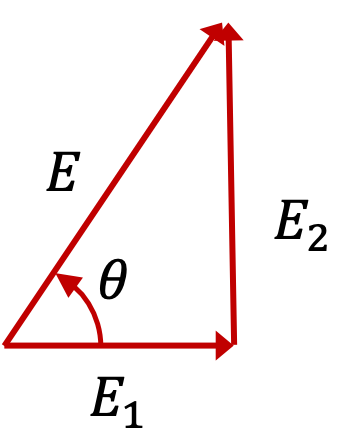Find the electric field at the origin.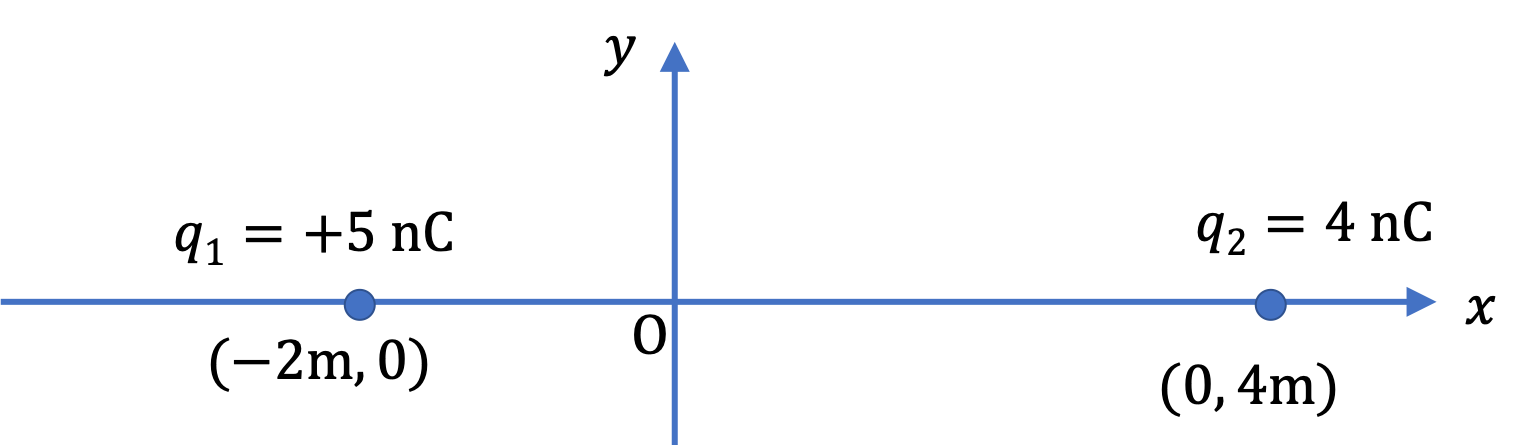Hint

The electric field of the two charges are in the opposite direction.

$9\text{ N/C}$ in the positive $x$ direction.

Solution

The following figure shows the electric field of the two charges at the origin are along the $x$ axis, but they are in the opposite direction. We will compute $x$ components of the two fields so that we can deal with their addition through the sign of the components.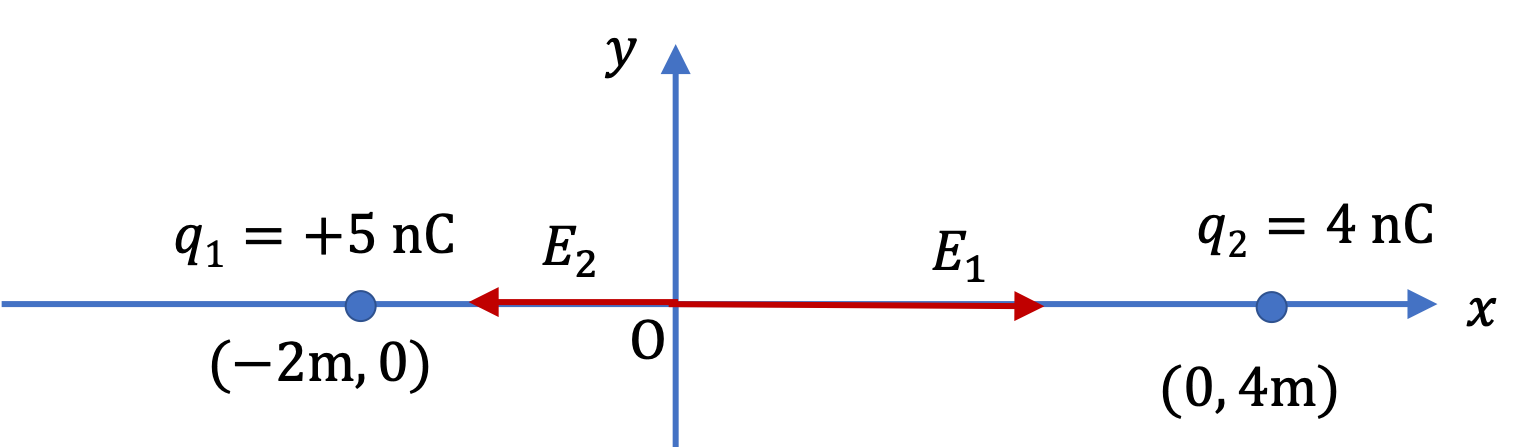The $x$ component of the fields are

\begin{align*} E_{1x} \amp = + k\dfrac{|q_1|}{r_1^2} = 9\times 10^{9} \times \dfrac{5\times 10^9}{2^2} = 11.25\text{ N/C}, \\ E_{2x} \amp = - k\dfrac{|q_2|}{r_2^2} = -9\times 10^{9} \times \dfrac{4\times 10^9}{4^2} = -2.25\text{ N/C}. \end{align*}

Therefore, $x$ component of the net electric field at origin is

\begin{equation*} E_x = E_{1x} + E_{2x} = 11.25 + (- 2.25) = 9\text{ N/C}. \end{equation*}

This is the only non-zero component of the field. Therefore, electric field at origin has magnitude $9\text{ N/C}$ and direction in the positive $x$ direction.

Find the magnitude and direction of the electric field at point P.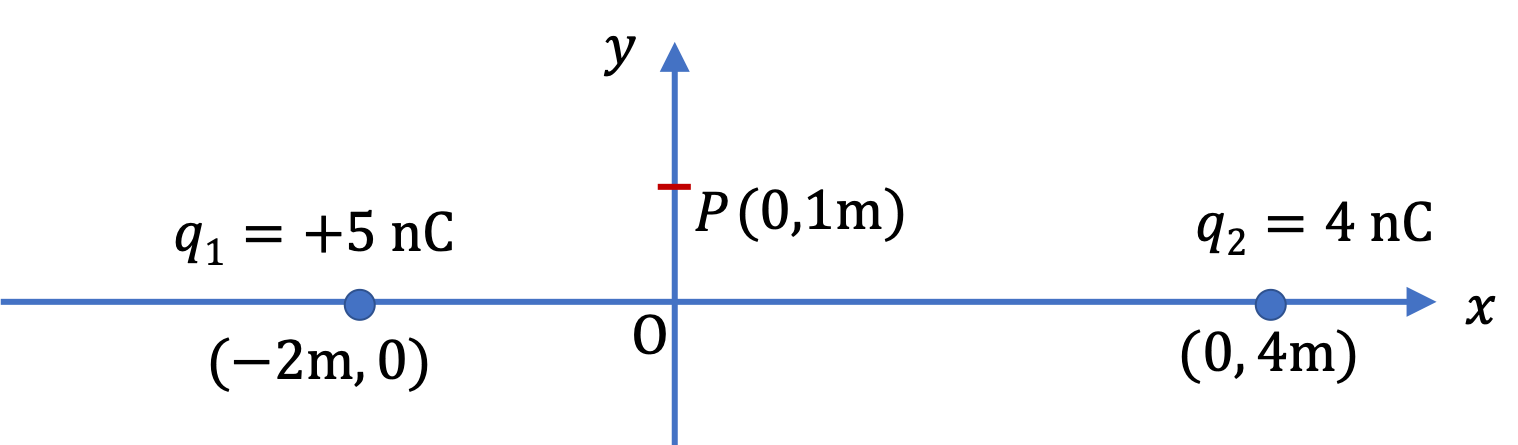Hint

Work with $x$ and $y$ components of electric fields from each charge.

$7.52\text{ N/C}$ counterclockwise at $37^\circ$ from the positive $x$ axis.

Solution

The following figure shows the electric field of the two charges are pointed in $xy$-plane. We will compute $x$ and $y$ components of the two fields so that we can deal with their addition through their components.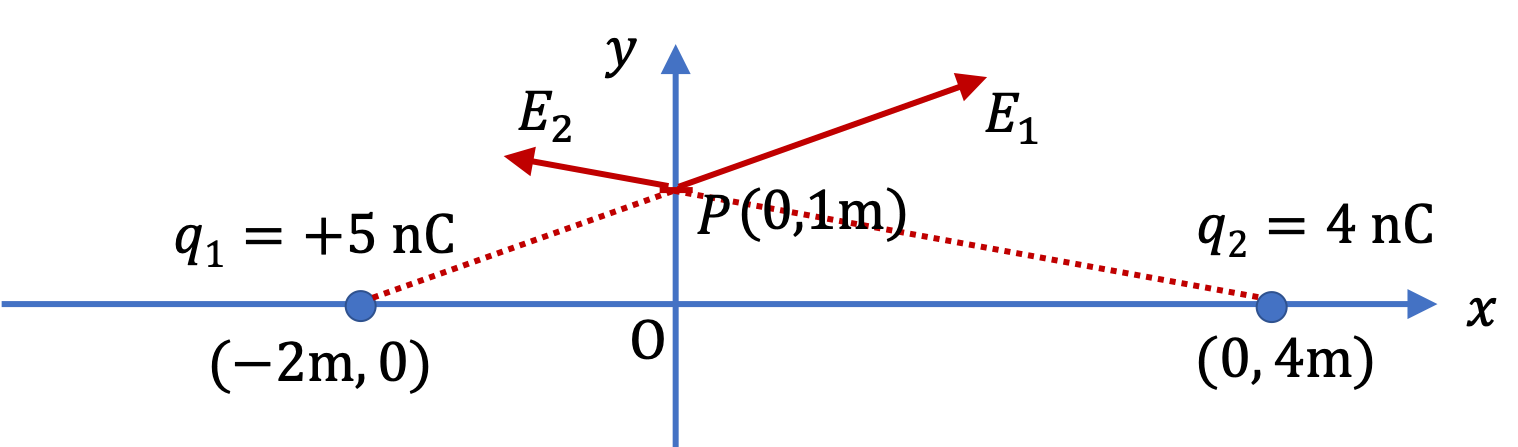The $x$ and $y$ components of electric field $\vec E_1$ are

\begin{align*} E_{1x} \amp = k\dfrac{q_1}{r_1^2} \dfrac{x_P-x_1}{r_1}, \\ E_{1y} \amp = k\dfrac{q_2}{r_2^2} \dfrac{y_P-y_1}{r_1}, \end{align*}

and similarly for charge $q_2\text{.}$ Computing the numerical values is easier by working out the parts of the formula first.

\begin{align*} r_1 \amp = \sqrt{ \left(x_P-x_1 \right)^2 + \left(y_P-y_1 \right)^2 } \\ \amp = \sqrt{ \left(0-(-2) \right)^2 + \left(1-0 \right)^2 } = \sqrt{ 5}\text{ m}. \\ r_2 \amp = \sqrt{ \left(x_P-x_2 \right)^2 + \left(y_P-y_2 \right)^2 } \\ \amp = \sqrt{ \left(0-(4) \right)^2 + \left(1-0 \right)^2 } = \sqrt{ 17}\text{ m}. \end{align*}

Therefore, the components of $\vec E_1$ will be

\begin{align*} E_{1x} \amp = 9\times 10^9\times\dfrac{5\times 10^{-9}}{ (\sqrt{5})^2 } \times \dfrac{0-(-2)}{\sqrt{5}} = 8.05\text{ N/C}, \\ E_{1y} \amp = 9\times 10^9\times\dfrac{5\times 10^{-9}}{ (\sqrt{5})^2 } \times \dfrac{1-0}{\sqrt{5}} = 4.02\text{ N/C}, \end{align*}

and those of $\vec E_2$ will be

\begin{align*} E_{2x} \amp = 9\times 10^9\times\dfrac{4\times 10^{-9}}{ (\sqrt{17})^2 } \times \dfrac{0-(4)}{\sqrt{17}} = -2.05\text{ N/C}, \\ E_{2y} \amp = 9\times 10^9\times\dfrac{4\times 10^{-9}}{ (\sqrt{17})^2 } \times \dfrac{1-0}{\sqrt{17}} = 0.51\text{ N/C}, \end{align*}

Therefore, the net electric field at P has the following components.

\begin{align*} E_x\amp = E_{1x} + E_{2x} = 8.05-2.05 = 6.00\text{ N/C}, \\ E_y\amp = E_{1y} + E_{2y} = 4.02+0.51 = 4.53\text{ N/C}, \end{align*}

The magnitude of electric field will be

\begin{equation*} E_P = \sqrt{ 6.00^2 + 4.53^2 } = 7.52\text{ N/C}, \end{equation*}

and the direction will be given by the counterclockwise angle $\theta$ from the positive $x$ axis,

\begin{equation*} \theta = \tan^{-1}\left(\dfrac{4.53}{6.00} \right) = 37^\circ. \end{equation*}

Four charges A( $+3\text{ nC}$ ), B( $-4\text{ nC}$ ), C( $+6\text{ nC}$ ) and D( $-9\text{ nC}$ ) are fixed to the successive corners of a square of side $5\ \mu\text{m}\text{.}$

Hint

Place A, B, C, and D on the axes and the center at the origin. Vectorially, add up the electric fields of the four charges.

Magnitude = $4.19\times 10^{12}\text {N/C}\text{,}$ Direction: $31^\circ$ clockwise below B-to-D direction.

Solution

First decision we make is how to place the charges so that our calculaiton is simplest. I found that if I place the charges on $x$ and $y$ coordinates with the center at the origin, the calculation works out quite simple.

The distance $r$ to the center of the square will be same for the four charges.

\begin{equation*} r = \frac{5}{\sqrt{2}} \ \mu\text{m}= 3.54 \ \mu\text{m}. \end{equation*}

The magnitudes of the electric field from the four charges will be

\begin{align*} E_P^{\textrm{by A}} \amp = 9\times 10^9 \times \frac{3\times 10^{-9}}{\left(3.54\times 10^{-6}\right)^2} = 2.15\times 10^{12}\ \textrm{N/C}\\ E_P^{\textrm{by B}} \amp = E_P^{\textrm{by A}} \times \frac{4}{3} = 2.87\times 10^{12}\ \textrm{N/C} \\ E_P^{\textrm{by C}} \amp = E_P^{\textrm{by A}} \times \frac{6}{3} = 4.31 \times 10^{12}\ \textrm{N/C}\\ E_P^{\textrm{by D}} \amp = E_P^{\textrm{by A}} \times \frac{9}{3} = 6.46 \times 10^{12}\ \textrm{N/C} \end{align*}

The directions of these electric fields are shown in the figure. With the choice of the $x$ and $y$ coordinates shown in the figure we find the $x$ - and $y$ -components of the net electric field at the center to be

\begin{align*} E_{x} \amp = \left( 2.87 - 6.46 \right)\times 10^{12}\ \textrm{N/C}\\ \amp = -3.59\times 10^{12}\ \textrm{N/C}\\ E_{y} \amp = \left( 4.31 - 2.15 \right)\times 10^{12}\ \textrm{N/C}\\ \amp = = 2.16\times 10^{12}\ \textrm{N/C} \end{align*}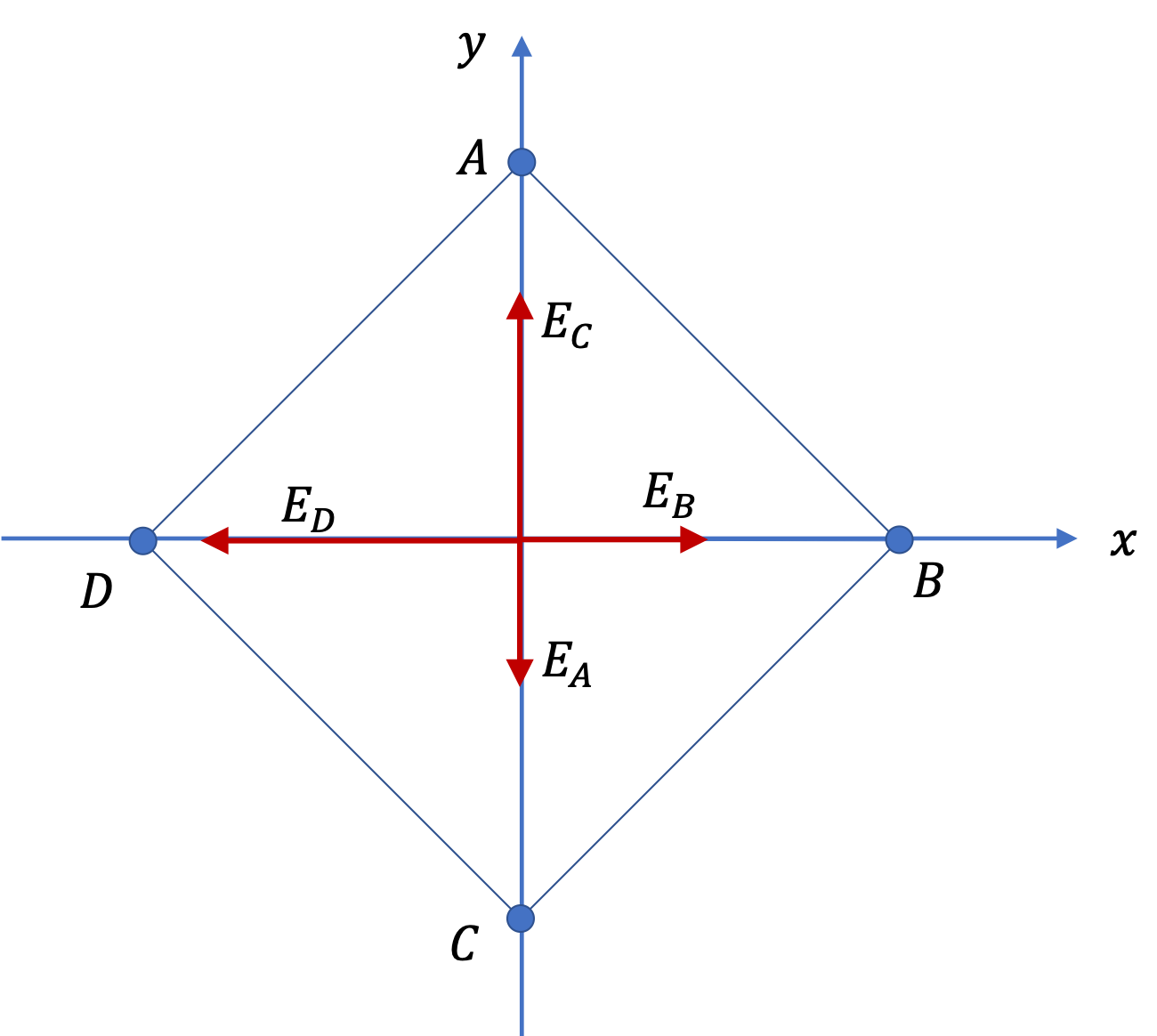The magnitude if the electric field will be

\begin{equation*} E= \sqrt{ 3.59^3 + 2.16^2 } \times 10^{12} = 4.19\times 10^{12}\text{ N/C}. \end{equation*}

Since point $(-3.59, 2.16)$ is in the second quadrant, the direction will be clockwise from the negative $x$axis

\begin{equation*} \theta = \tan^{-1} \left( \dfrac{2.16}{-3.59}\right) = -31^{\circ}. \end{equation*}

Two charges $+q$ and $-q$ are placed on the $y$-axis at $y = \dfrac{d}{2}$ and $y = -\dfrac{d}{2}$ respectively. The two equal-but-opposite charge system is called a dipole. Find the electric field at an arbitrary point P $(x,y,0)$ in the $xy$-plane.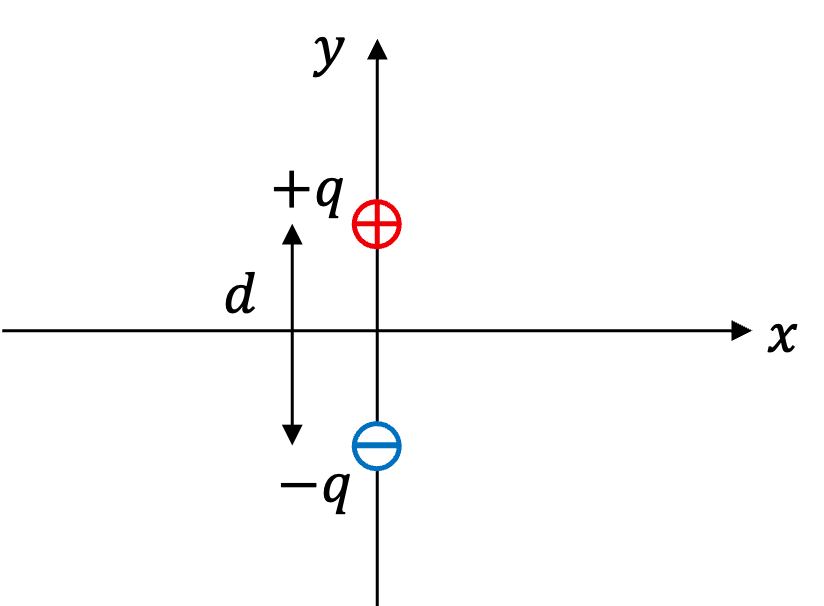Hint

Use superposition of two electric fields.

See solution.

Solution

The distances $r_{-}$ and $r_{+}$ of the two charge

The net electric field will be a vector sum of the electric fields from each charge. Let us denote the electric field of $+q$ by $\vec E_1$ and that of $-q$ by $\vec E_2$ . We can write the Cartesian components of these electric fields at P as follows.

\begin{align*} E_{1x} \amp = \frac{k\:q\:x}{ \left[ x^2 + (y-d/2)^2 \right]^{3/2} }\\ E_{1y} \amp = \frac{k\:q\:(y-d/2)}{ \left[x^2 + (y-d/2)^2 \right]^{3/2} }\\ E_{2x} \amp = \frac{-k\:q\:x}{ \left[x^2 + (y+d/2)^2 \right]^{3/2} }\\ E_{2y} \amp = \frac{-k\:q\:(y+d/2) }{ \left[x^2 + (y+d/2)^2 \right]^{3/2} } \end{align*}

The Cartesian components of the net electric field $\vec E$ at the field point P will be

\begin{align*} E_x \amp = E_{1x} + E_{2x},\\ E_y \amp = E_{1y} + E_{2y}. \end{align*}

From these we will get the magnitude and direction as angle as

\begin{align*} E \amp = \sqrt{E_x^2 + E_y^2},\\ \theta \amp = \tan^{-1}\left( \dfrac{E_y}{E_x} \right). \end{align*}